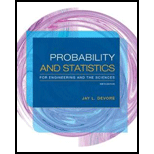# Let t = the amount of sales tax a retailer owes the government for a certain period. The article “Statistical Sampling in Tax Audits” ( Statistics and the Law , 2008: 320–343) proposes modeling the uncertainty in t by regarding it as a normally distributed random variable with mean value µ and standard deviation σ (in the article, these two parameters are estimated from the results of a tax audit involving n sampled transactions). If a represents the amount the retailer is assessed, then an under-assessment results if t &gt; a and an over-assessment results if a &gt; t . The proposed penalty (i.e., loss) function for over- or under-assessment is L( a , t ) = t − a if t &gt; a and = k ( a − t ) if t ≤ a ( k &gt; 1 is suggested to incorporate the idea that over-assessment is more serious than under-assessment). a. Show that a * = µ + σ Φ −1 (1/( k + 1)) is the value of a that minimizes the expected loss, where Φ −1 is the inverse function of the standard normal cdf. b. If k = 2 (suggested in the article), µ = $100,000, and σ =$10,000, what is the optimal value of a , and what is the resulting probability of over-assessment?### Probability and Statistics for Eng...

9th Edition
Jay L. Devore
Publisher: Cengage Learning
ISBN: 9781305251809### Probability and Statistics for Eng...

9th Edition
Jay L. Devore
Publisher: Cengage Learning
ISBN: 9781305251809

#### Solutions

Chapter 4, Problem 110SE
Textbook Problem

## Expert Solution

### Want to see the full answer?

Check out a sample textbook solution.

### Want to see this answer and more?

Experts are waiting 24/7 to provide step-by-step solutions in as fast as 30 minutes!*

*Response times vary by subject and question complexity. Median response time is 34 minutes and may be longer for new subjects.